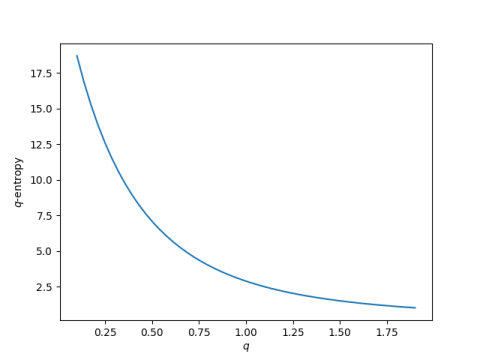# Generalizing Shannon entropy with q-logs

The most common way to quantify entropy is Shannon entropy. That’s what people usually mean when they say “entropy” without further qualifications. A while back I wrote about Renyi entropy as a generalization of Shannon entropy. This time I’ll explore a different generalization called q-log entropy, a.k.a. Tsallis entropy.

The definition of Shannon entropy includes the logarithms of probabilities. If we replace these logarithms with a generalization of logarithms, we have a generalization of Shannon entropy.

## q-logarithms

The generalization of logarithm we want to look at for this post is the q-logarithm. The natural logarithm is given byand we can generalize this to the q-logarithm by definingAnd so ln1 = ln.

## q-logarithm entropy

We can define q-log entropy (a.k.a. Tsallis entropy) by replacing natural log with q-log. However we run into a minor issue.

Shannon entropy (in nats, see ) can be defined as eitherorThese are equivalent becauseHoweverunless q = 1.

On the other hand, it’s easy to show thatAnd so we could use either lnq(1/p) or -lnq(p) in our definition of q-log entropy.The two definitions are not equivalent unless q = 1, but they are related by## Example

To see q-log entropy in action, we’ll plot the entropy of the English alphabet as q varies.This plot was made using English letter frequencies from here and the following Python code.

    def lnq(x, q):
if q == 1:
return np.log(x)
t = 1 - q
return (x**t - 1) / t

def shannon_entropy(ps, base=2):
s = sum(p*np.log(1/p) for p in ps)
return s/np.log(base)

def qlog_entropy(ps, q):
return sum(p*lnq(1/p, q) for p in ps)


The Shannon entropy of the English alphabet is 2.8866 nats . The q-log entropy is greater than that for q less than 1, and smaller than that for q greater than 1.

## Related posts

 Shannon entropy is usually defined in terms of logarithms base 2, i.e. entropy is usually measured in bits. If we change the base of the logarithm, we only multiply the result by a constant. So we can define Shannon entropy using natural logarithms, resulting in entropy measured in “nats.” More on bits, dits, and nats here.

 Or 4.1645 bits. See  above for the difference between bits and nats.

## 3 thoughts on “Generalizing Shannon entropy with q-logs”

1. There don’t appear to be many applications of Renyi or Tsallis entropy, compared to Shannon entropy, which is a shame because they seem to be more interesting:
http://shape-of-code.coding-guidelines.com/2017/11/15/a-la-carte-entropy/

My theory is that Shannon entropy is the fall-back topic for researchers to wave their arms about when they cannot think of anything else to say: